# How to Create a User Defined Function in Excel VBA?

• Last Updated : 16 Dec, 2021

A function is a collection of code.  As a developer, we very often need a custom function (User-defined function) in our projects. Here we explain how to create a UDF (user-defined function) to count/return the Number of vowels in a given string using Excel VBA.

Example Custom Function Syntax:

```Function Name: countVowel()
Input parameter: an excel cell
Output: Return an integer (Number of vowels)```

### Implementation:

Follow the below steps to create a User-defined Function in Excel VBA:

Step 1: Open an excel file, To create user-defined function “countVowel()” function.

Step 2: Press Alt + F11 – to open Visual Basic Editor.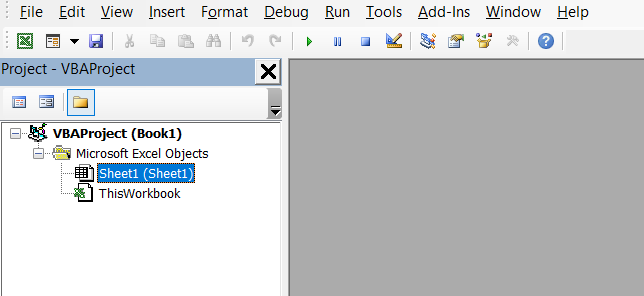Step 3: Click Insert >> Module – which will add a new module as in Img1.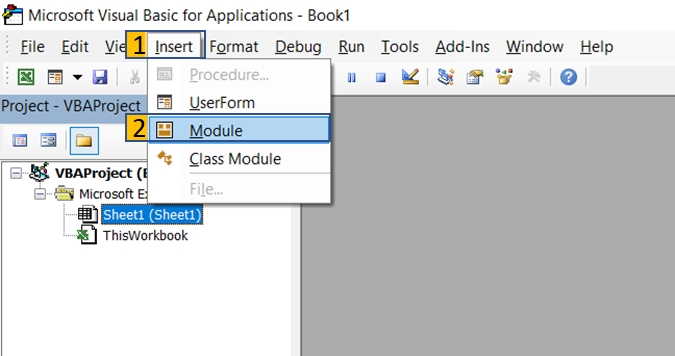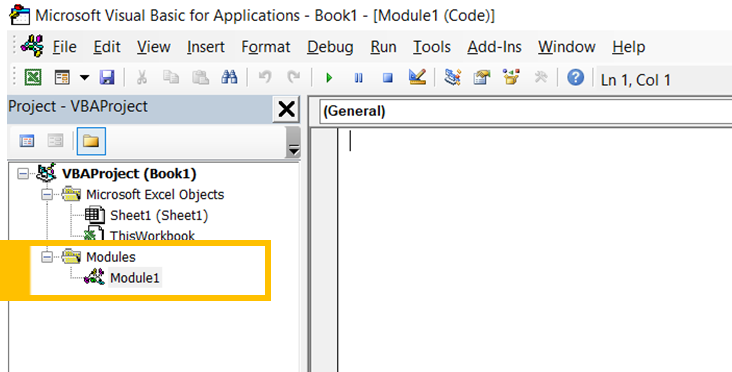Img 1

Step 4: Type the below code in “code window”.

• Function name with parameter Range
`Function countVowel(rg As Range)`
• Iterate each character of a given string and check the character is vowel or not.  If it is vowel character increase vowelCount = vowelCount +1
```For i = 1 To Len(rg.Value)
textValue = UCase(Mid(rg.Value, i, 1))
If textValue Like "[AEIOU]" Then
vowelCount = vowelCount + 1
End If
Next i```
• Return number of vowels.
```countVowel = vowelCount
End Function ```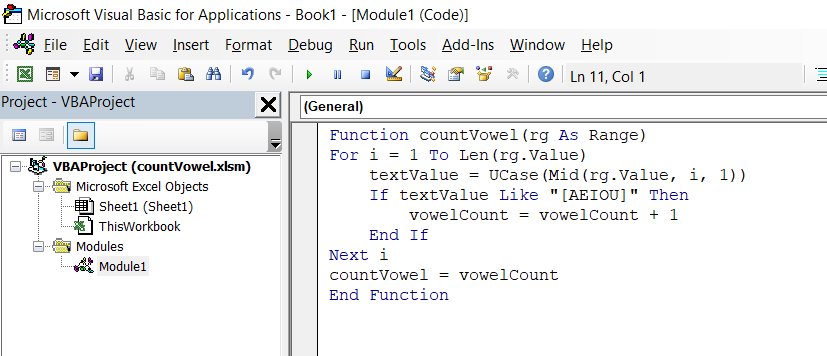Step 5: Save your Excel workbook as Excel Macro-Enabled Workbook (*.xlsm)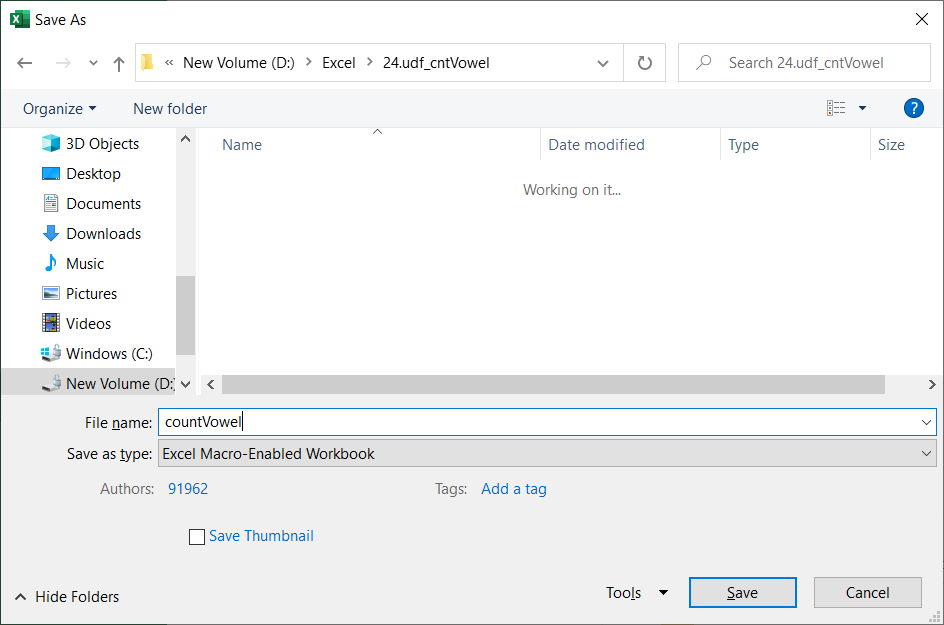Step 6: Type “Customer” in cell “A1” and “Vowels_Count” in cell “B1” as header.

• Fill names in cells A2:A6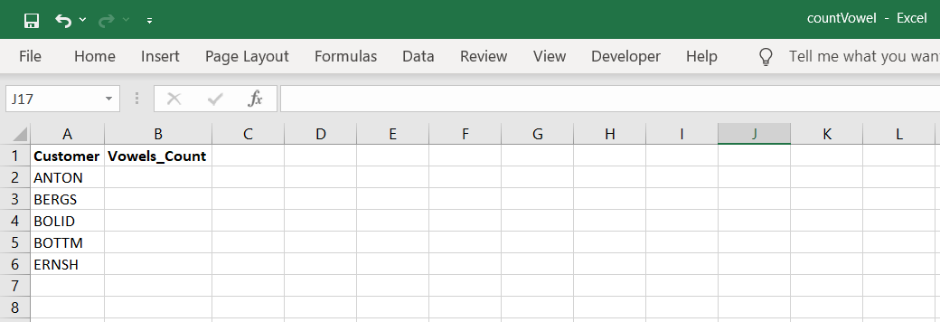Step 7: Write below formula in cell “B2” (Img 2) and drag it to fill “B2:B6” (Img 3)

`=countVowel(A2)`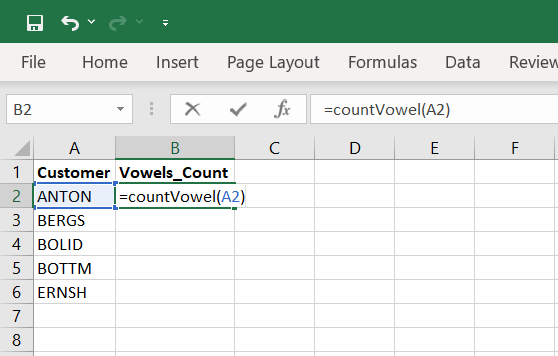Img 2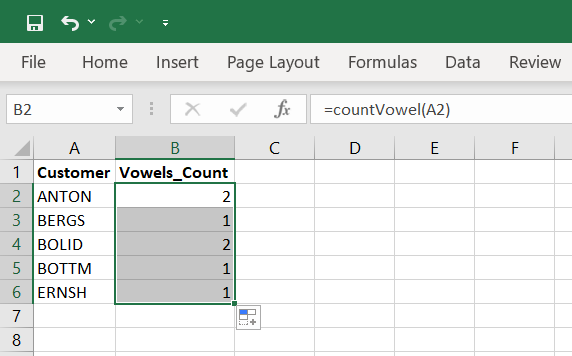Img 3

My Personal Notes arrow_drop_up